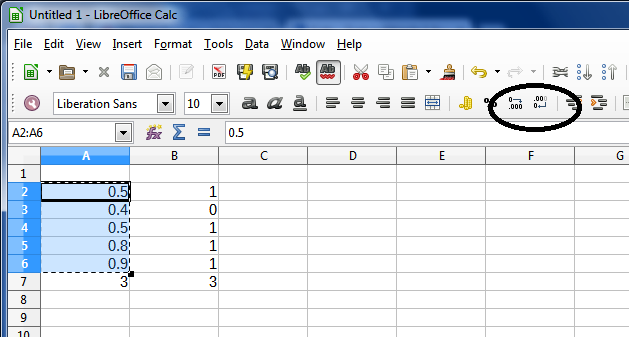# Calc - Sum subtracts 1 from total

I am using Sum to add the total of a row. Instead of the total, sum actually gives me a number that is less 1. For example, I have a row that should add up to 6. The total it is giving me is 5. What did I do wrong?

You will have to supply more information, precisely what you have done and what numbers you are using.

One possibility may be that the actual values of the numbers in the cells are not what you are seeing.

For example, 0.5+0.4+0.5+0.8+0.9=3

However, if these if I change the decimal display to a whole number they appear to be:
1+0+1+1+1. However, the sum of these numbers will be 3 because of the actual values as shown above.

The image below shows the buttons I used to increase or decrease the decimals.